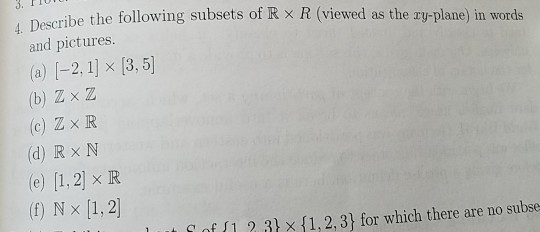# Question 4. Describe the following su bsets of R × R (viewed as the xy-plane) in words and pictures (b) Z×Z (c) Z x R (d) R x N (e) [1,2] × R (f) N x [1, 2 t f 112 3 X 1,2, 31 for which there are no subseHelp with this would be appreciated, thanks

Transcribed Image Text: 4. Describe the following su bsets of R × R (viewed as the xy-plane) in words and pictures (b) Z×Z (c) Z x R (d) R x N (e) [1,2] × R (f) N x [1, 2 t f 112 3 X 1,2, 31 for which there are no subse
More
Transcribed Image Text: 4. Describe the following su bsets of R × R (viewed as the xy-plane) in words and pictures (b) Z×Z (c) Z x R (d) R x N (e) [1,2] × R (f) N x [1, 2 t f 112 3 X 1,2, 31 for which there are no subse